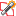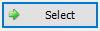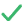# Sets from Cluster Analysis

The Sets from Cluster Analysisoption allows you to quickly determine data clusters (joint sets) on the stereonet using an algorithm based on fuzzy Cluster Analysis. The Sets from Cluster Analysis option requires the user to first manually pick the approximate center of a data cluster and then the fuzzy cluster algorithm will automatically determine which poles belong to the nearest corresponding joint set within a specified maximum radius.

Once the poles belonging to a set have been determined, the mean plane orientation and set statistics are calculated in exactly the same way as for other methods of set creation (e.g., Set Windows).

To create Sets using Cluster Analysis:

1. Select Sets from Cluster Analysisbutton from the toolbar or the Sets menu.
2. In the Sets From Cluster Analysis dialog:
• Set the Limit Cluster Size to Cone with Maximum Angle value. The maximum cone angle limits the extent of a data cluster (i.e., all poles in the set will fall within the specified cone angle, assuming that the cone axis is located at the mean pole orientation). A default cone angle (30 degrees) is in effect, you can change this as required.
• If you want to generate a Symbolic Plot of Set ID after the Set(s) have been determined, select the Show Sets with Symbolic Plot when Finished checkbox.
3. When you are ready to pick Sets, choose the Selectbutton in the dialog. The dialog will close and you will be prompted to pick the approximate location of cluster centers.
4. NOTE: You should be viewing a Contour Plot of pole density so that you can easily identify data clusters on the stereonet.

5. As you move the mouse cursor over the stereonet, you will notice that a cone of the specified maximum radius follows the cursor. This cone gives you a visual estimate of the maximum extent of the Set(s) which will be created.
6. Using the pole density contours as a guide, choose the approximate center of a data cluster for which you would like to create a Set. A temporary cone will be displayed at the clicked on location.
7. You may repeat the previous step for each data cluster that you wish to analyze. A temporary cone will be added at each clicked on location.
8. When you have finished clicking on all desired set locations, press ENTER or right-click and select Donefrom the popup menu.
9. The fuzzy cluster algorithm will then automatically determine a set corresponding to each selected location, and the cone angle.
10. Set Windows are automatically drawn for each set determined by the Cluster Analysis.
11. A symbolic pole plot of Set ID will automatically be generated if the Show Sets with Symbolic Plot when Finished checkbox was selected in step 2.

It is important to note the following regarding the Sets from Cluster Analysis option.

## Seed Point

When you select the approximate center of a data cluster (step 5), the point which you select is used as a "seed" point for the fuzzy cluster algorithm. This point is NOT (necessarily) the final center (mean) of the Set, since this is determined by the fuzzy cluster algorithm. You can verify this as follows:

1. When you select a set center location (step 5), select a point which is off center (i.e. not exactly at the maximum pole concentration).
2. You will notice that the fuzzy cluster algorithm will locate the "true" center of the data cluster, even if the initial seed point is some distance from the true center.
3. For any given set, experiment with how far the seed point can be from the true center, before the fuzzy cluster algorithm chooses a different data cluster. This will also be a function of the maximum cone angle entered in step 2.

If you would like to use a different Maximum Cone Radius for different Sets, you will have to repeat the above procedure for each different radius you would like to use.

## Set Statistics from Cluster Analysis

Once a data cluster has been determined by the fuzzy cluster algorithm, the Mean Set Plane orientation and Set statistics are calculated using exactly the same method as sets determined by other methods available in Dips (i.e., the various Set Window options). In other words, for a given grouping of poles, the mean orientation and set statistics will be exactly the same, regardless of the method used to create the Set in Dips.

## Set Windows from Cluster Analysis

After a Cluster Analysis, you will notice that, in general, the Set Windows which are automatically created after the Cluster Analysis, are "stepped" (i.e., the windows are automatically fitted to only encompass the poles which belong to the Set, according to the Fuzzy Cluster Analysis).

Unlike the curvilinear 4-sided Set Windows created with the Add Set Window option, the Set Windows determined from Cluster Analysis cannot be edited once they have been created. They can be deleted like regular Set Windows, but they cannot be graphically edited like the 4-sided Set Windows.

## References

The fuzzy cluster algorithm used in Dips is based on work presented in the following paper. Click the link to view the paper:

Fuzzy Cluster Algorithm for the Automatic Identification of Joint Sets, R.E. Hammah, J.H. Curran, International Journal of Rock Mechanics & Mining Sciences, Vol.35, No. 7, pp.889-905, 1998.

In its implementation in Dips some modifications were made for the purpose of incorporating the option into the Dips program, but the basic algorithm is based on this work. Currently in Dips, Cluster Analysis is based solely on pole orientation. In future versions of Dips other non-orientation information (e.g., joint type, roughness, surface, etc.) may be considered in the analysis in order to identify joint sets based on orientation and other attributes.